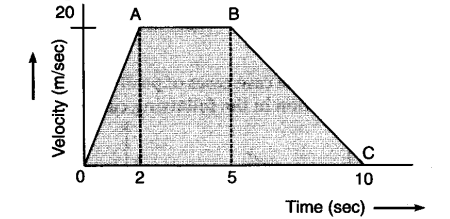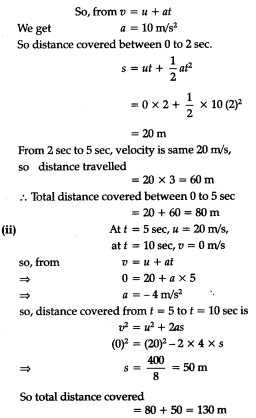# The velocity-time graph of an object moving along a straight line is as shown :

The velocity-time graph of an object moving along a straight line is as shown :Calculate distance covered by object between:
(i) t = 0 to t = 5 sec.
(ii) f = 0 to # = 10 sec.

(i) Velocity at t = 0, u = 0
Velocity at t = 2 sec, v = 20 m/s